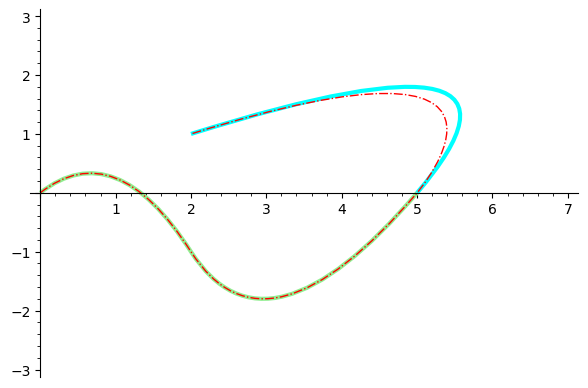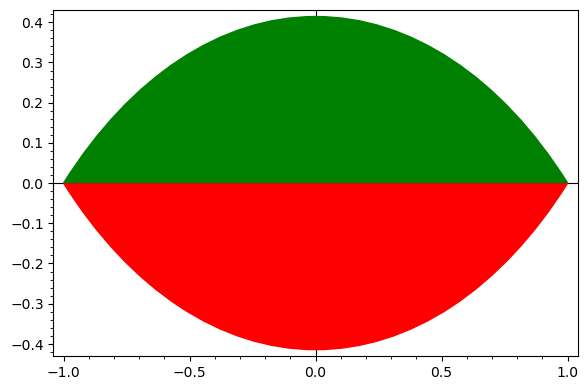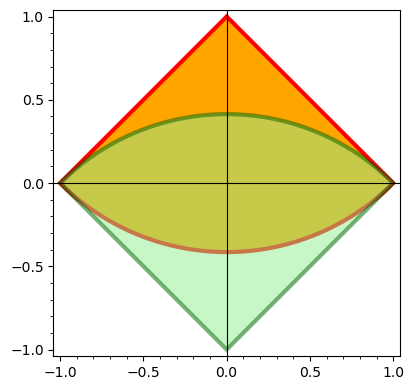# Concatenate bezier paths?

I have two bezier paths path1 and path2 (constructed using arc(...)). They have the same beginning point and end point, and I'd like to join them into a single path and fill the interior. What's the right way to do this? If I just try to concatenate the lists path1.path and path2.path and then call bezier_curve() and the result, it generates an error when I try to display it.

Here is a simple failed attempt.

arc1 = arc((0,1),sqrt(2),sector=(5*pi/4,7*pi/4))
arc2 = arc((0,-1),sqrt(2),sector=(pi/4,3*pi/4))

path1 = arc1.bezier_path()
path2 = arc2.bezier_path()

combo = bezier_path(path1.path+path2.path)
combo.show()

edit retag close merge delete

This question is strongly connected with this other one: Filling a hyperbolic polygon. Read my answer in this related question to see a method to concatenate Bézier paths.

Sort by » oldest newest most votedYou can't concatenate Bézier paths by simply concatenating the corresponding lists. For example, let B1 and B2 be the Bézier paths generated by the lists L1=[[p1,c1,p2], [c2,p3]] and L2=[[p3,c3,p4]], and let C be the Bézier path associated to L1+L2=[[p1,c1,p2], [c2,p3], [p3,c3,p4]]. The concatenation of B1and B2 is formed by three quadratic Bézier curves. However, C is composed by two quadratic Bézier curves and a cubic one. Note that [p3,c3,p4] in L1+L2 is interpreted as a curve starting at p3 and ending at p4 which has two control points p3 and c3, so it is a cubic Bézier curve. This situation can be clearly seen in the plot generated by the following code, where B1, B2 and C are colored in green, blue and red respectively:

L1 = [[(0,0),(1,1),(2,-1)],[(3,-3),(5,0)]]
L2 = [[(5,0),(7,3),(2,1)]]
B1 = bezier_path(L1,rgbcolor="lightgreen",thickness=3)
B2 = bezier_path(L2,rgbcolor="cyan",thickness=3)
C = bezier_path(L1+L2,rgbcolor="red",linestyle="-.")
show(B1+B2+C)In your case there is the additional problem that, due to rounding errors, both paths have different endpoints:

sage: path1
Bezier path from (-1.0000000000000004, 3.3306690738754696e-16) to (1.0, -2.220446049250313e-16)
sage: path2
Bezier path from (1.0000000000000002, 0.0) to (-1.0, 2.220446049250313e-16)


It seems that you just want to fill the region limited by both paths. You can achieve this by changing some graphic options as follows:

arc1 = arc((0,1),sqrt(2),sector=(5*pi/4,7*pi/4))
arc2 = arc((0,-1),sqrt(2),sector=(pi/4,3*pi/4))

path1 = arc1.bezier_path()
path2 = arc2.bezier_path()

opts = path1.options()
opts.update({"fill": True, "rgbcolor": "red"})
path1.set_options(opts)

opts = path2.options()
opts.update({"fill": True, "rgbcolor": "green"})
path2.set_options(opts)

show(path1 + path2, frame=True)


This is the output:Hope this helps.

Edited (10/12/2020): An alternative route to get similar plots could be to define a function like the following one:

def circular_arc(center, radius, sector=(0,pi), segment=True,
plot_points=50, **kwargs):
step = (sector-sector)/(plot_points-1)
for t in [sector..sector,step=step]]
if(not segment): pts.append(center)
return polygon(pts,**kwargs)


This function has a syntax similar to that of arc. It plots the circular segment determined by the arc if segment=True, or the corresponding circular sector if segment=False. With this function at hand, the following code yields almost exactly the same output as above:

arc1 = circular_arc((0,1), sqrt(2), sector=(5*pi/4,7*pi/4), color="red")
arc2 = circular_arc((0,-1), sqrt(2), sector=(pi/4,3*pi/4), color="green")
show(arc1 + arc2, frame=True, aspect_ratio=golden_ratio)


Just for the purpose of comparison, let us show what happens with segment=False:

arc1 = circular_arc((0,1), sqrt(2), sector=(5*pi/4,7*pi/4), segment=False,
color="orange", edgecolor="red", thickness=3)
arc2 = circular_arc((0,-1), sqrt(2), sector=(pi/4,3*pi/4), segment=False,
color="lightgreen", edgecolor="darkgreen",
thickness=3, alpha=0.5)
show(arc1 + arc2, frame=True)more

Nice! I think the idea is to draw hyperbolic polygons in the disc model. See

So you would want to fill a region that is bounded by several circle arcs but in a concave rather than convex way. For instance:

arc((1, -1), 1, sector((pi/2, pi))
arc((-1, -1), 1, sector((0, pi/2))
arc((-1, 1), 1, sector((-pi/2, 0))
arc((1, 1), 1, sector((-pi, -pi/2))


The link doesn't work. Did you mean this question? https://ask.sagemath.org/question/54314/filling-a-hyperbolic-polygon/ I see. Perhaps it would worthy to add fill as an allowed option for the bezier_path method, or, even better, for the arc graphics primitive.

Sorry there was a typo in the link I gave (fixed now). You correctly figured out what page I meant.

Yes, a fill option for Bezier paths would be nice! Would you open a ticket and contribute that?

Thinking more carefully about this question, perhaps it is not such a good idea to add the fill option. It is ambiguous what should be filled: the circular sector or the circular segment determined by the arc? There is also alternative simple ways to get the same results. See the edit to my answer.

Filling a path that closes up should make sense.

That operation is available in PostScript, SVG, TikZ, ...

Possibly in matplotlib too?

For paths that do not close up, add a final segment to close it up and fill.